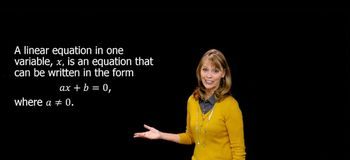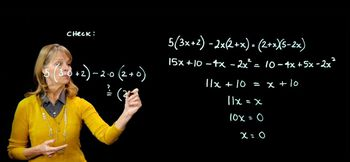Start typing, then use the up and down arrows to select an option from the list.
6:15 minutes
Problem 55
Textbook Question

# Exercises 41–60 contain rational equations with variables in denominators. For each equation, a. write the value or values of the variable that make a denominator zero. These are the restrictions on the variable. b. Keeping the restrictions in mind, solve the equation. 3/(x + 2) + 2/(x - 2) = 8/(x + 2)(x - 2)Verified Solution
This video solution was recommended by our tutors as helpful for the problem above.
56views